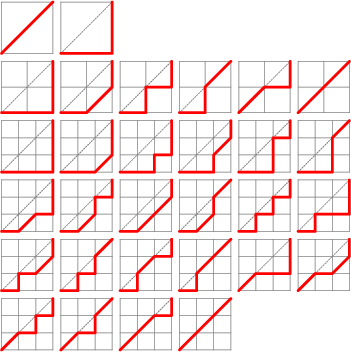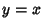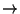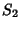## Schröder NumberThe Schröder numberis the number of Lattice Paths in the Cartesian plane that start at (0, 0), end at, contain no points above the line, and are composed only of steps (0, 1), (1, 0), and (1, 1), i.e.,,, and. The diagrams illustrating the paths generating,, andare illustrated above. The numbersare given by the Recurrence Relationwhere, and the first few are 2, 6, 22, 90, ... (Sloane's A006318). The Schröder Numbers bear the same relation to the Delannoy Numbers as the Catalan Numbers do to the Binomial Coefficients.

See also Binomial Coefficient, Catalan Number, Delannoy Number, Lattice Path, Motzkin Number, p-Good Path

References

Sloane, N. J. A. Sequence A006318/M1659 in An On-Line Version of the Encyclopedia of Integer Sequences.'' http://www.research.att.com/~njas/sequences/eisonline.html and Sloane, N. J. A. and Plouffe, S. The Encyclopedia of Integer Sequences. San Diego: Academic Press, 1995.